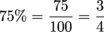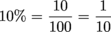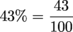Study Guide

# Converting Percents to Fractions

## Converting Percents to Fractions

We've got one more conversion trick for ya: zapping percents into fractions.25% means 25 out of 100. When we reduce that fraction, it simplifies to. Like we can see in the image above, 25% is the same thing as a quarter of the whole.

To convert a percent to a fraction, place the percent number over 100, and reduce the fraction when possible. That's it.

Example time. To convert 75% to a fraction, we stick 75 over 100 and reduce it like there's no tomorrow. To do that, we divide the top and bottom by 25.One of the easier percents to write as a fraction is 10%. Again, we throw 10 in the numerator and 100 in the denominator, then reduce.Of course, some percentages can't be reduced at all when we stick 'em into fraction form. For example, to turn 43% into a fraction, just put 43 up top and 100 down low.That fraction doesn't reduce, so we're done. High fives all around.